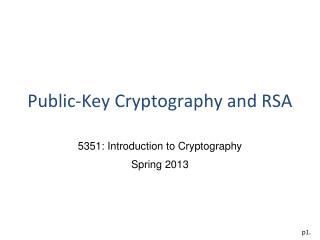# Public-Key Cryptography and RSA - PowerPoint PPT PresentationDownload PresentationPublic-Key Cryptography and RSA

Public-Key Cryptography and RSADownload Presentation## Public-Key Cryptography and RSA

- - - - - - - - - - - - - - - - - - - - - - - - - - - E N D - - - - - - - - - - - - - - - - - - - - - - - - - - -
##### Presentation Transcript

1. Public-Key Cryptography and RSA 5351: Introduction to Cryptography Spring 2013

2. Public-Key Cryptography • Also known as asymmetric-key cryptography. • Each user has a pair of keys: a public key and a private key. • The public key is used for encryption. • The key is known to the public. • The private key is used for decryption. • The key is only known to the owner.

3. Why Public-Key Cryptography? • Developed to address two main issues: • key distribution • digital signatures • Invented by Diffie & Hellman in 1976.

4. Modular Arithmetic

5. The Chinese Remainder Problem • A problem described in an ancient Chinese arithmetic book, Sun TzeSuanChing, by Sun Tze (around 300AD, author of The Art of War). • Problem: We have a number of objects, but we do not know exactly how many. If we count them by threes we have two left over. If we count them by fives we have three left over. If we count them by sevens we have two left over. How many objects are there?

6. Example: Chinese remainder theorem

7. Algorithms

8. The RSA Cryptosystem RSA Encryption RSA Digital Signature

9. Attacks on RSA